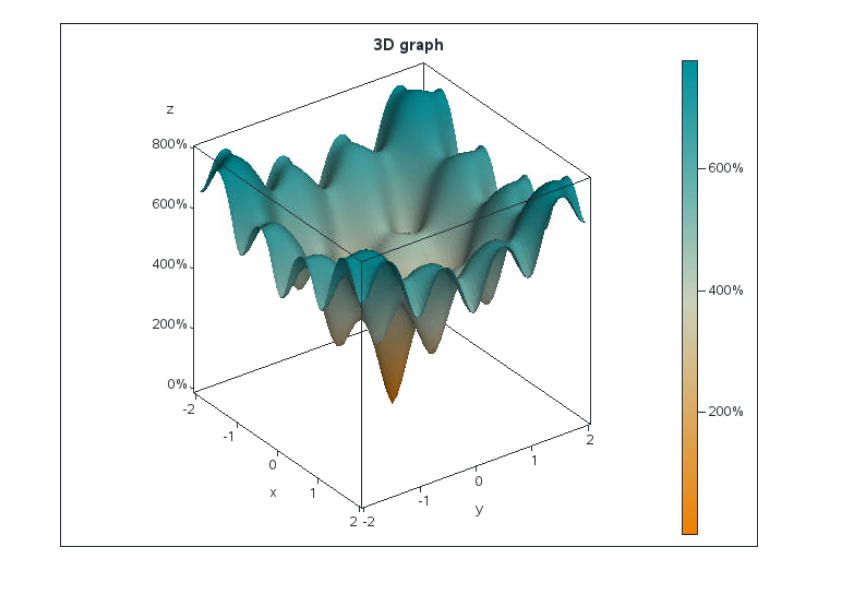## display a function

Hello everybody,

I want to display a graph with the ackley function.

``````/*ackley function*/
y=(-20)*(exp(-0.2*sqrt(0.5*(x**2+x**2))))-(exp(0.5*(cos(2*4*atan(1)*x)+cos(2*4*atan(1)*x))))+exp(1)+20;``````

For this, I will use g3d, g3grid and maybe gcontour.

The first step is to create the data statement in order to have x, y and z.

I have : z=y*x

then the form of x and y must be similar than the form of the table sashelp.lake

But, I need x and y from an uniform law.

I have code the beginning in IML:

``````proc iml;
xmax=2;
xmin=-2;
range=xmax-xmin;
Y=j(range,1,0);
X=j(range,1,0);
X1=j(range*range,1,0);
do i=1 to range;
call randgen(u,'uniform');
Y[i,1]=xmin+u*range;
end;

do i=1 to range/2;
y=y//y;
end;

do i=1 to range;
call randgen(u1,'uniform');
X[i,1]=xmin+u1*range;
end;

do i=1 to range;
do n=1 to range*range;
X1[n,1]=X[i,1];
end;
end;
print X1;
run;
quit;``````

At the end, I have the correct Y but, I don't get the correct form of X which is like :

range times the first value, range times the second, etc.

1 ACCEPTED SOLUTION

Accepted Solutions

## Re: display a function

``````proc iml;
_x=do(-2,2,0.05);
_y=_x;
x=expandgrid(_x,_y);

z=j(nrow(x),3,.);
do i=1 to nrow(x);
z[i,1]=(-20)*(exp(-0.2*sqrt(0.5*(x[i,1]**2+x[i,2]**2))))-
(exp(0.5*(cos(2*4*atan(1)*x[i,1])+cos(2*4*atan(1)*x[i,2]))))+exp(1)+20;
z[i,2:3]=x[i,];
end;
create in_graph from z [colname={'z' 'x' 'y'}];
append from z;
close;
run;

/* From Rick's post at:                                     */
/* http://blogs.sas.com/content/iml/create-surface-plot-sas */
proc template;                        /* surface plot with continuous color ramp */
define statgraph SurfaceTmplt;
dynamic _KMS _AGE _Z _Title;              /* dynamic variables */

begingraph;
entrytitle _Title;                   /* specify title at run time (optional) */
layout overlay3d / tilt=30
xaxisopts=(label="x")
yaxisopts=(label="y" linearopts=(tickvaluelist=(-2 -1 0 1 2 )))
zaxisopts=(label="z" linearopts=(tickvalueformat=xval.));

surfaceplotparm x=_KMS y=_AGE z=_Z /  /* specify variables at run time */

name="surface"
surfacetype=fill
colormodel=threecolorramp      /* or =twocolorramp */
colorresponse=_Z
reversecolormodel=true;
continuouslegend "surface";

endlayout;
endgraph;
end;
run;

/* And... Render the Hat! */
proc sgrender data=in_graph template=SurfaceTmplt;
dynamic _KMS='X' _AGE='Y' _Z='Z' _Title="3D graph";
format Z percent7.1;
run;``````5 REPLIES 5

## Re: display a function

``````proc iml;
_x=do(-2,2,0.05);
_y=_x;
x=expandgrid(_x,_y);

z=j(nrow(x),3,.);
do i=1 to nrow(x);
z[i,1]=(-20)*(exp(-0.2*sqrt(0.5*(x[i,1]**2+x[i,2]**2))))-
(exp(0.5*(cos(2*4*atan(1)*x[i,1])+cos(2*4*atan(1)*x[i,2]))))+exp(1)+20;
z[i,2:3]=x[i,];
end;
create in_graph from z [colname={'z' 'x' 'y'}];
append from z;
close;
run;

/* From Rick's post at:                                     */
/* http://blogs.sas.com/content/iml/create-surface-plot-sas */
proc template;                        /* surface plot with continuous color ramp */
define statgraph SurfaceTmplt;
dynamic _KMS _AGE _Z _Title;              /* dynamic variables */

begingraph;
entrytitle _Title;                   /* specify title at run time (optional) */
layout overlay3d / tilt=30
xaxisopts=(label="x")
yaxisopts=(label="y" linearopts=(tickvaluelist=(-2 -1 0 1 2 )))
zaxisopts=(label="z" linearopts=(tickvalueformat=xval.));

surfaceplotparm x=_KMS y=_AGE z=_Z /  /* specify variables at run time */

name="surface"
surfacetype=fill
colormodel=threecolorramp      /* or =twocolorramp */
colorresponse=_Z
reversecolormodel=true;
continuouslegend "surface";

endlayout;
endgraph;
end;
run;

/* And... Render the Hat! */
proc sgrender data=in_graph template=SurfaceTmplt;
dynamic _KMS='X' _AGE='Y' _Z='Z' _Title="3D graph";
format Z percent7.1;
run;``````## Re: display a function

Thank you very much. Very helpful.

## Re: display a function

Wow, except for vectorizing the Ackley function, these programs are virtually identical, right down to the references! I don't think I've ever seen that before for a long program.

## Re: display a function

If you want to create points on a uniform grid, use the EXPANDGRID function. If you want to create a surface plot, see Create a surface plot in SAS - The DO Loop

``````%let dx = 0.1;
%let dy = 0.1;

proc iml;
xmin=-2;
xmax=2;
xLin = do(xmin, xmax, &dx);

ymin=-2;
ymax=2;
yLin = do(ymin, ymax, &dy);

grid = expandgrid(xLin, yLin);
x = grid[,1];
y = grid[,2];

/*ackley function*/
pi = constant('pi');
z = -20*(exp(-0.2*sqrt(0.5*(x##2+y##2)))) -
(exp(0.5*(cos(2*pi*x)+cos(2*pi*y)))) + exp(1) + 20;

create Ackley var {x y z};
append;
close;
QUIT;

title "Quick Visualization";
proc sgplot data=Ackley;
scatter x=x y=y / colorresponse=z markerattrs=(symbol=SquareFilled size=14)
colormodel=ThreeColorRamp;
run;
/* https://blogs.sas.com/content/iml/2015/10/12/create-surface-plot-sas.html */
proc template;                        /* surface plot with continuous color ramp */
define statgraph SurfaceTmplt;
dynamic _X _Y _Z _Title;              /* dynamic variables */
begingraph;
entrytitle _Title;                   /* specify title at run time (optional) */
layout overlay3d;
surfaceplotparm x=_X y=_Y z=_Z /  /* specify variables at run time */
name="surface"
surfacetype=fill
colormodel=threecolorramp      /* or =twocolorramp */
colorresponse=_Z;              /* prior to 9.4m2, use SURFACECOLORGRADIENT= */
continuouslegend "surface";
endlayout;
endgraph;
end;
run;
proc sgrender data=Ackley template=SurfaceTmplt;
dynamic _X='X' _Y='Y' _Z='Z' _Title="Ackley Function";
run;
``````

Thank you, Rick.
From The DO Loop